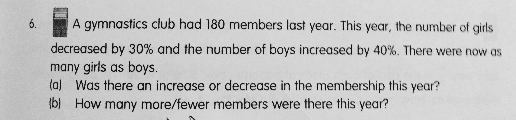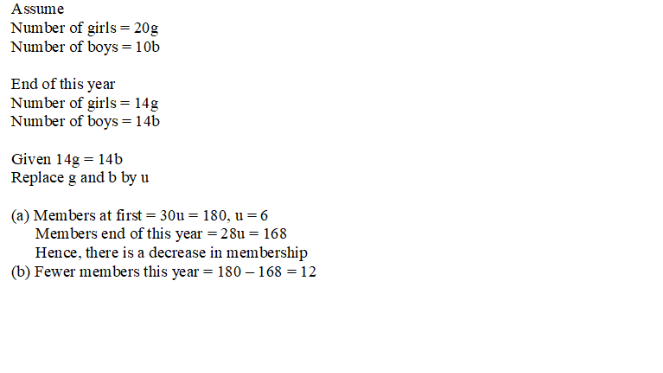# QuestionAnyone help me with attached question

Thanks

(a)
last year —
girls : 100u
boys : 100p

100u + 100p ——- 180

this year —
girls : 100u – 30u = 70u
boys : 100p + 40p = 140p

70u ——- 140p
1u ——- 2p

200p + 100p = 300p ——- 180
1p ——- 0.6
140p ——- 140 x 0.6 = 84
84 x 2 = 168 (decrease)

(b)
180 + 168 = 12

Ans : (a) decrease; (b) 12 less members.

0 Replies 1 Like ✔Accepted Answer0 Replies 1 Like

Here’s how i explain it.

a) if the no. of G has gone down, no. of B has gone up and they are now equal.

We originally had G + B = 180

We now have 7/10G = 14/10B, if we multiply both sides by 10,

7/10G = 14/10B

7G = 14B

G = 2B

This means there are half as many boys as girls. Since G + B = 180, we have 120 Girls and 60 Boys.

New no. of students = 7/10G + 14/10B = 84 + 84= 168

So no. of students has decreased

There were 180-168 = 12 less members this year

If anyone is interested in cheap primary/secondary maths tuition, I am a new young private maths tutor please look at my profile.

0 Replies 1 Like